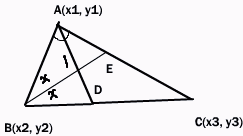Name: ___________________Date:___________________

 Email us to get an instant 20% discount on highly effective K-12 Math & English kwizNET Programs!

High School Mathematics - 29.17 Incentre of a Triangle

 To find the coordinates of the incentre of a triangle whose vertices are given Definition: The point of concurrence of the internal bisectors of the angles of a triangle is called the incentre and is generally denoted by I. Let A(x1, y1), B(x2, y2) and C(x3, y3) be the vertices of the triangle. Let AD and BE, the bisectors of the angles A and B meet in I.AD bisects angleBAC of triangle ABC Hence BD/DC = AB/BC = c/b D divides BC internally in the ratio c:b Hence the coordinates of D are [(cx3+bx2)/(c+b), (cy3 + by2)/(c+b)] Now, BI bisects angle ABD of triangle ABD Hence AI/ID = AB/BD = c/BD----equation 1 But BD/DC = c/b(Proved above) Hence BD/(BD+ DC) = c/(c+b) BD/BC = c/(c+b) Hence BD = ca/(c+b)(because BC = a) From equation 1 AI/ID = c/ca/(c+b) = (c+b)/a This shows that I divides AD internally in the ratio c+b:a The coordinates of I are x = {(c+b)[(cx3+bx2)/(c+b)]+ax1}/(c+b)+a = (ax1+bx2+cx3)/(a+b+c) y = {(c+b)[(cy3+by2)/(c+b)] + ay1}/(c+b) + a = (ay1+by2+cy3)/(a+b+c) Directions: Solve the following.Name: ___________________Date:___________________

High School Mathematics - 29.17 Incentre of a Triangle

 Q 1: Find the coordinates of the incentre of the triangle whose vertices are (7,-36), (7,20) and (-8,0)(0,-1)(0,1)(1,0)(-1,0) Q 2: Find the incentre of the triangle formed by (-4,1), (-1,2) and (0,5)(1,2)(1,-2)(-2,1)(2,-1) Q 3: If (0,1), (1,1)and (1,0) are the mid points of the sides of the triangle, find the incentre.(2,2)(2,-1)(1,2)(2-2Ö2, 2-2Ö2) Q 4: Find the coordinates of the incentre of the triangle whose vertices are (4,-2), (-2,4) and (5,5)1,-35/2, 5/22,-15,5 Question 5: This question is available to subscribers only! Question 6: This question is available to subscribers only!